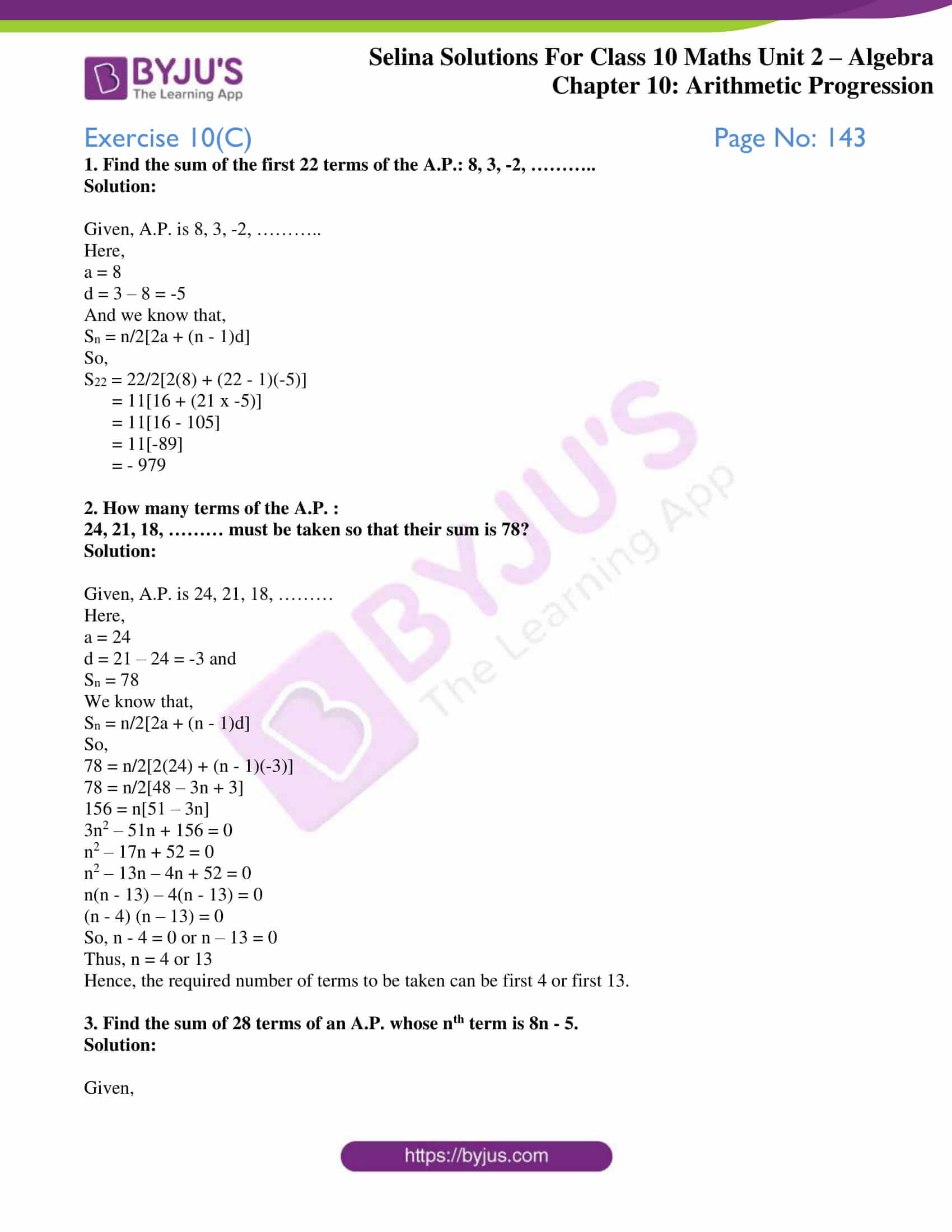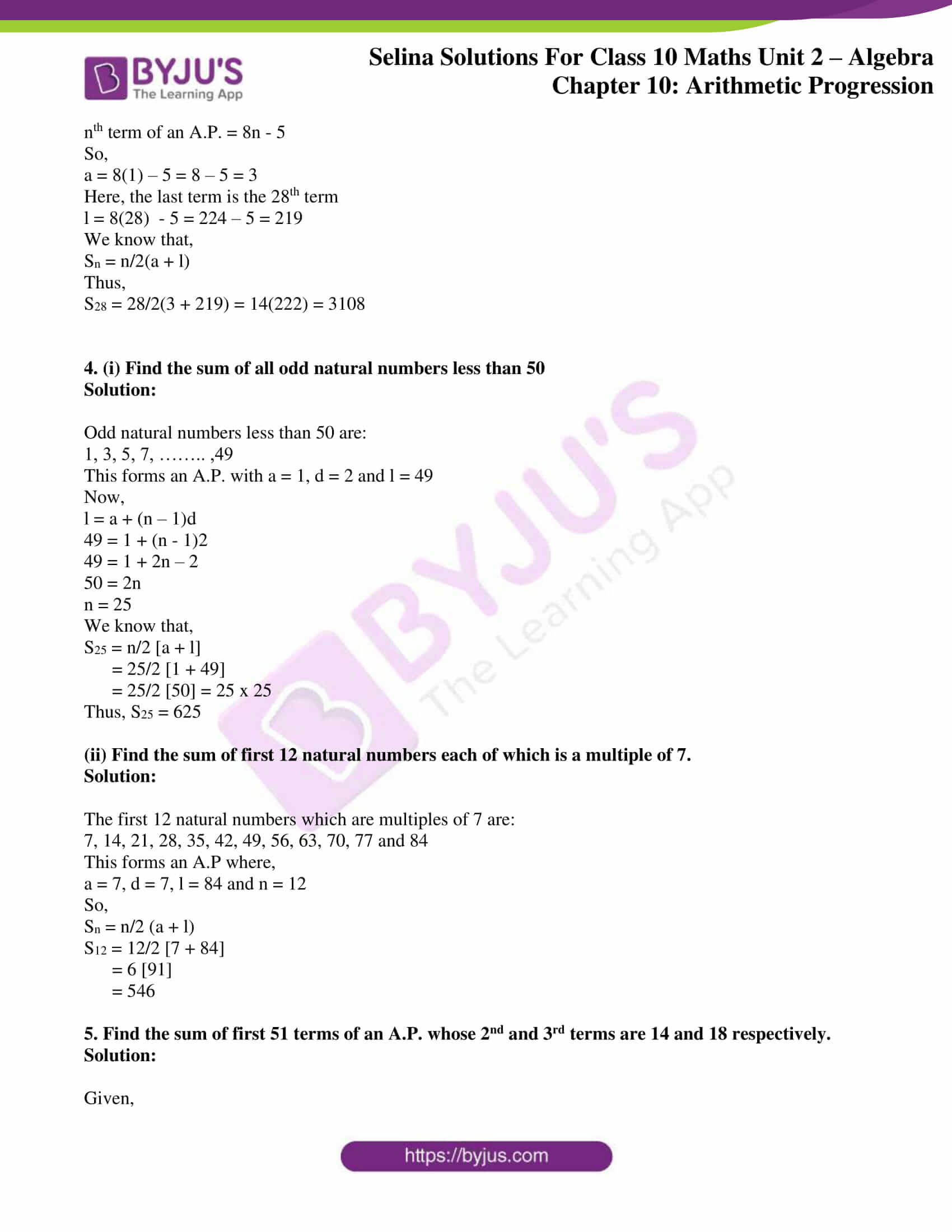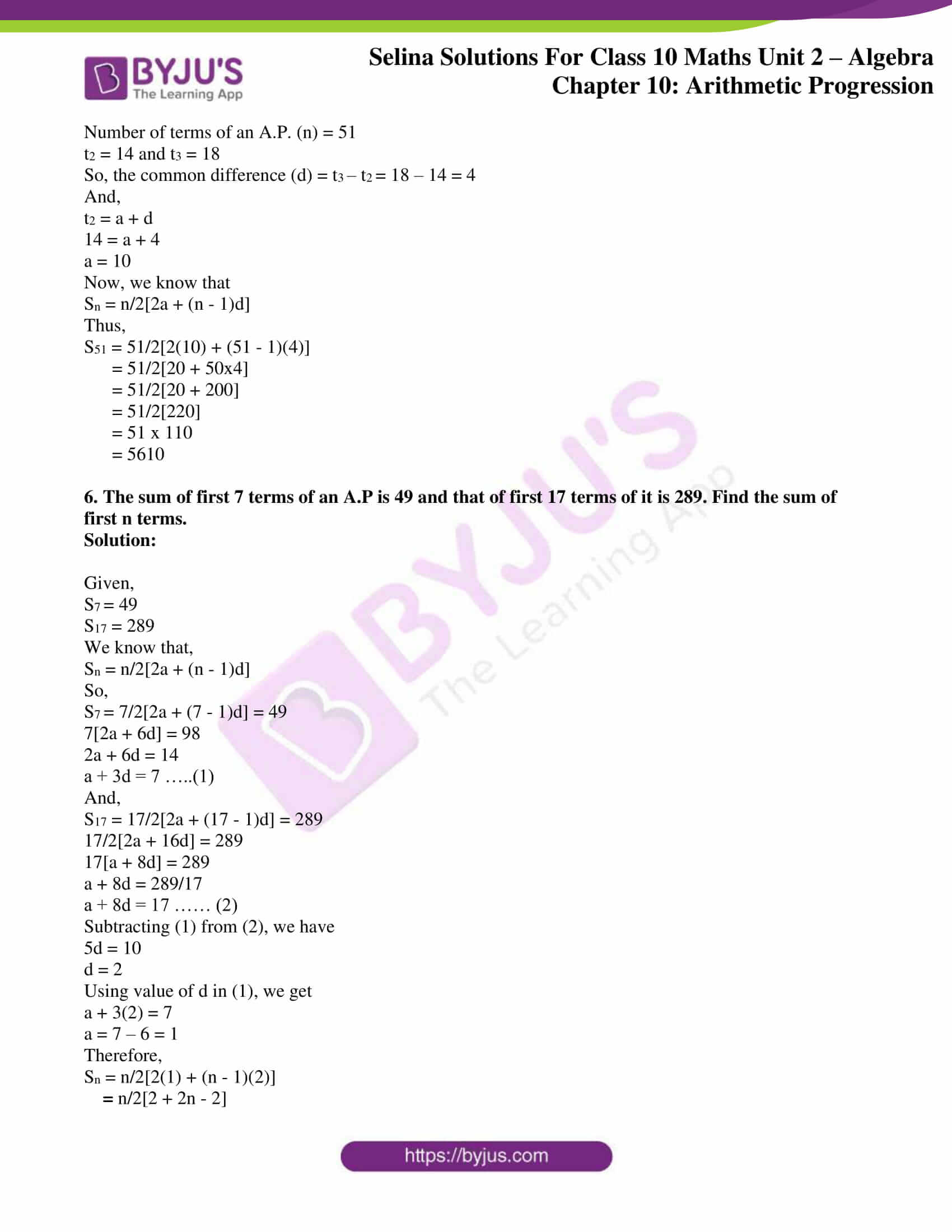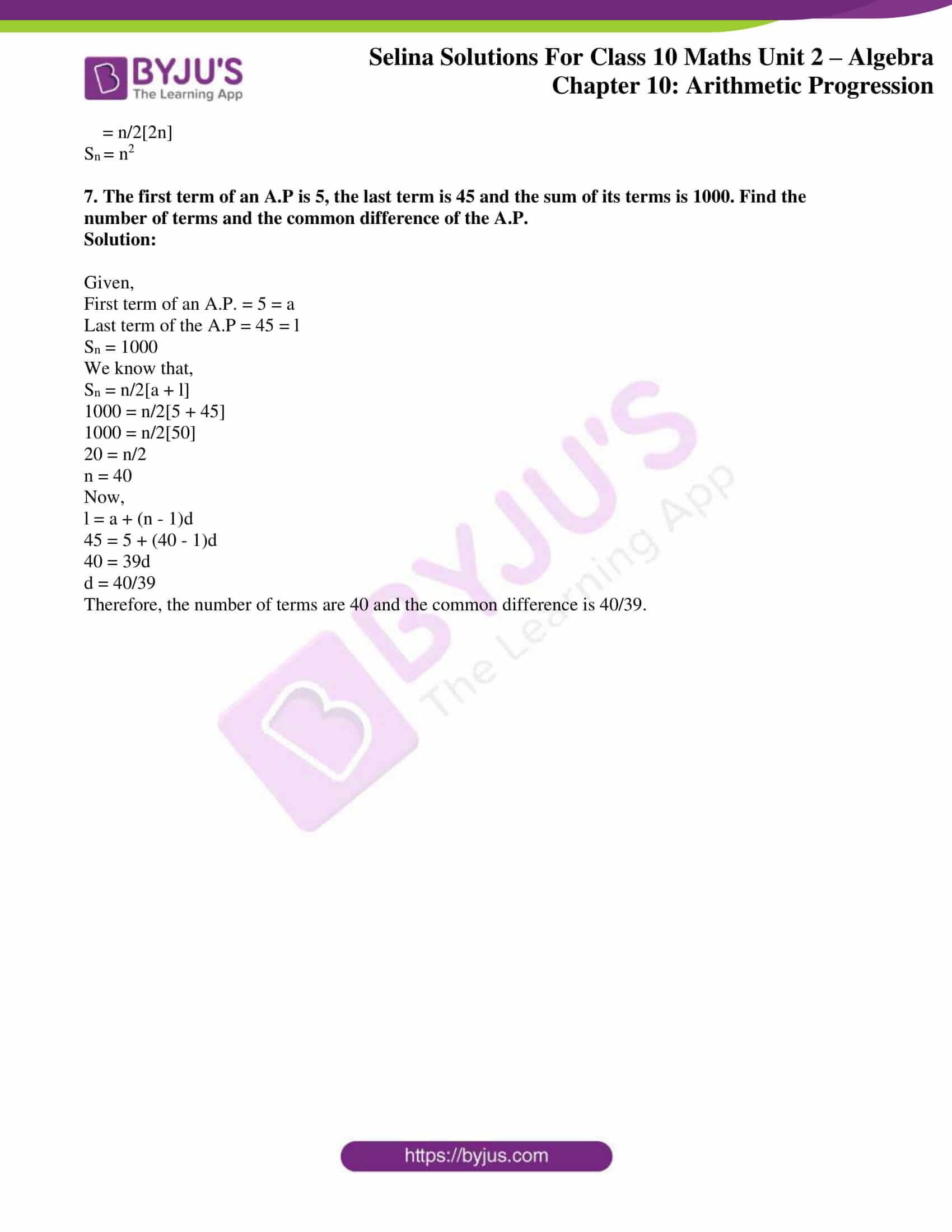# Selina Solutions Concise Maths Class 10 Chapter 10 Arithmetic Progression Exercise 10(C)

The concept of finding the sum of n terms of an A.P. is tested in this exercise. Students with uncleared doubts regarding solving problems of this Chapter can refer to Selina Solutions for Class 10 Maths. Furthermore, the precise answers to this exercise are available in the Selina Solutions Concise Maths for Class 10 Chapter 10 Arithmetic Progression Exercise 10(C), PDF which is given in the links below.

## Selina Solutions Concise Maths Class 10 Chapter 10 Arithmetic Progression Exercise 10(C) Download PDF### Access Selina Solutions Concise Maths Class 10 Chapter 10 Arithmetic Progression Exercise 10(C)

#### Exercise 10(C) Page No: 143

1. Find the sum of the first 22 terms of the A.P.: 8, 3, -2, ………..

Solution:

Given, A.P. is 8, 3, -2, ………..

Here,

a = 8

d = 3 – 8 = -5

And we know that,

Sn = n/2[2a + (n – 1)d]

So,

S22 = 22/2[2(8) + (22 – 1)(-5)]

= 11[16 + (21 x -5)]

= 11[16 – 105]

= 11[-89]

= – 979

2. How many terms of the A.P. :

24, 21, 18, ……… must be taken so that their sum is 78?

Solution:

Given, A.P. is 24, 21, 18, ………

Here,

a = 24

d = 21 – 24 = -3 and

Sn = 78

We know that,

Sn = n/2[2a + (n – 1)d]

So,

78 = n/2[2(24) + (n – 1)(-3)]

78 = n/2[48 – 3n + 3]

156 = n[51 – 3n]

3n2 – 51n + 156 = 0

n2 – 17n + 52 = 0

n2 – 13n – 4n + 52 = 0

n(n – 13) – 4(n – 13) = 0

(n – 4) (n – 13) = 0

So, n – 4 = 0 or n – 13 = 0

Thus, n = 4 or 13

Hence, the required number of terms to be taken can be first 4 or first 13.

3. Find the sum of 28 terms of an A.P. whose nth term is 8n – 5.

Solution:

Given,

nth term of an A.P. = 8n – 5

So,

a = 8(1) – 5 = 8 – 5 = 3

Here, the last term is the 28th term

l = 8(28) – 5 = 224 – 5 = 219

We know that,

Sn = n/2(a + l)

Thus,

S28 = 28/2(3 + 219) = 14(222) = 3108

4. (i) Find the sum of all odd natural numbers less than 50

Solution:

Odd natural numbers less than 50 are:

1, 3, 5, 7, …….. ,49

This forms an A.P. with a = 1, d = 2 and l = 49

Now,

l = a + (n – 1)d

49 = 1 + (n – 1)2

49 = 1 + 2n – 2

50 = 2n

n = 25

We know that,

S25 = n/2 [a + l]

= 25/2 [1 + 49]

= 25/2  = 25 x 25

Thus, S25 = 625

(ii) Find the sum of first 12 natural numbers each of which is a multiple of 7.

Solution:

The first 12 natural numbers which are multiples of 7 are:

7, 14, 21, 28, 35, 42, 49, 56, 63, 70, 77 and 84

This forms an A.P where,

a = 7, d = 7, l = 84 and n = 12

So,

Sn = n/2 (a + l)

S12 = 12/2 [7 + 84]

= 6 

= 546

5. Find the sum of first 51 terms of an A.P. whose 2nd and 3rd terms are 14 and 18 respectively.

Solution:

Given,

Number of terms of an A.P. (n) = 51

t2 = 14 and t3 = 18

So, the common difference (d) = t3 – t2 = 18 – 14 = 4

And,

t2 = a + d

14 = a + 4

a = 10

Now, we know that

Sn = n/2[2a + (n – 1)d]

Thus,

S51 = 51/2[2(10) + (51 – 1)(4)]

= 51/2[20 + 50×4]

= 51/2[20 + 200]

= 51/2

= 51 x 110

= 5610

6. The sum of first 7 terms of an A.P is 49 and that of first 17 terms of it is 289. Find the sum of first n terms.

Solution:

Given,

S7 = 49

S17 = 289

We know that,

Sn = n/2[2a + (n – 1)d]

So,

S7 = 7/2[2a + (7 – 1)d] = 49

7[2a + 6d] = 98

2a + 6d = 14

a + 3d = 7 …..(1)

And,

S17 = 17/2[2a + (17 – 1)d] = 289

17/2[2a + 16d] = 289

17[a + 8d] = 289

a + 8d = 289/17

a + 8d = 17 …… (2)

Subtracting (1) from (2), we have

5d = 10

d = 2

Using value of d in (1), we get

a + 3(2) = 7

a = 7 – 6 = 1

Therefore,

Sn = n/2[2(1) + (n – 1)(2)]

= n/2[2 + 2n – 2]

= n/2[2n]

Sn = n2

7. The first term of an A.P is 5, the last term is 45 and the sum of its terms is 1000. Find the number of terms and the common difference of the A.P.

Solution:

Given,

First term of an A.P. = 5 = a

Last term of the A.P = 45 = l

Sn = 1000

We know that,

Sn = n/2[a + l]

1000 = n/2[5 + 45]

1000 = n/2

20 = n/2

n = 40

Now,

l = a + (n – 1)d

45 = 5 + (40 – 1)d

40 = 39d

d = 40/39

Therefore, the number of terms are 40 and the common difference is 40/39.

### Access other exercises of Selina Solutions Concise Maths Class 10 Chapter 10 Arithmetic Progression

Exercise 10(A) Solutions

Exercise 10(B) Solutions

Exercise 10(D) Solutions

Exercise 10(E) Solutions

Exercise 10(F) Solutions